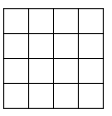# Original 9251

If the length of the square pad tent is reduced by 6 cm, its area will be reduced by 2.76 dm2. Specify the side length of the original and reduced pads.

a =  26 cm
b =  20 cm

### Step-by-step explanation:Did you find an error or inaccuracy? Feel free to write us. Thank you!

Tips for related online calculators
Are you looking for help with calculating roots of a quadratic equation?
Do you have a linear equation or system of equations and looking for its solution? Or do you have a quadratic equation?
Do you want to convert length units?

#### Grade of the word problem:

We encourage you to watch this tutorial video on this math problem: